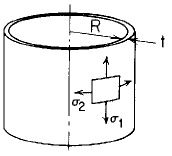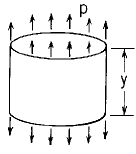Related Resources: pressure-vessel

### Cylinder Uniform Axial Load Equation and Calculator

Cylindrical Pressure Vessel Uniform Axial Load Equation and Calculator.

Per. Roarks Formulas for Stress and Strain for membrane stresses and deformations in thin-walled pressure vessels.

 Cylindrical( R / t ) > 10 Uniform Loading axial loadWhen:
R / t > 10

Meridional StressCircumferential or Hoop StressChange in height dimension yRotation of a meridian from its unloaded position
ψ = 0

Where used:
E = Modulus of Elasticity (lbs/in2)
v = Poisson's ratio
σ1,2 = stress, (lbs/in2)
t = Cylinder thickness, (in)
y = Length of cylinder, (in)
p = Applied unit load (lbs/in)
ψ = Rotation of a meridian from its unloaded position, positive when that meridional rotation represents an increase in ΔR when y or θ increases;

Reference:

Roarks Formulas for Stress and Strain, 7th Edition